# Don't use Laplace method Don't use Laplace method Don't use Laplace method Don't use Laplace meth...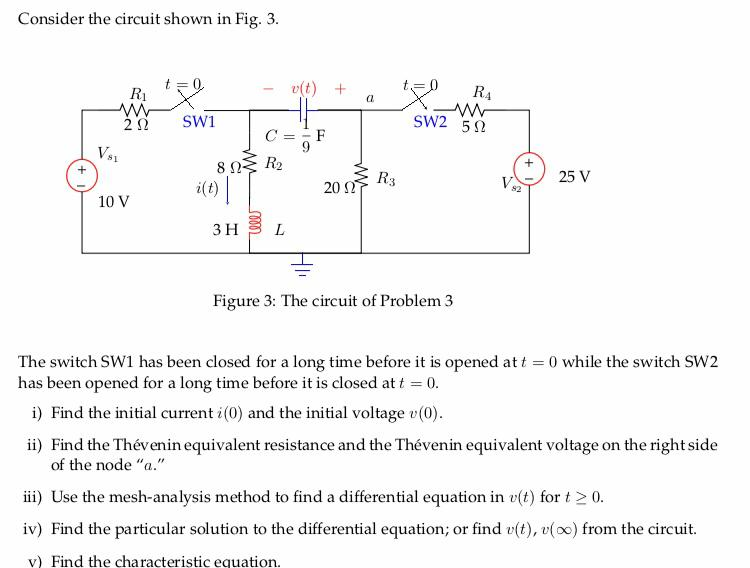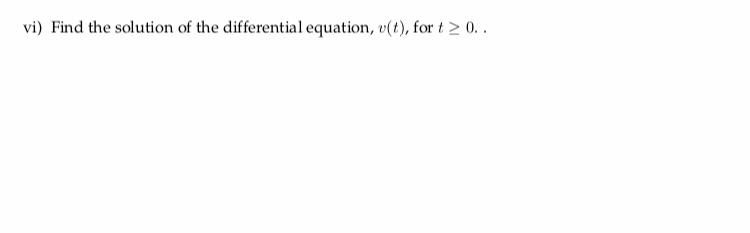Don't use Laplace method

Don't use Laplace method

Don't use Laplace method

Don't use Laplace method

Don't use Laplace method

Consider the circuit shown in Fig. 3 22 SW1 SW2 5Ω CF 25 V 20 S 10 V 3 H BL Figure 3: The circuit of Problem3 The switch SW1 has been closed for a long time before it is opened att has been opened for a long time before it is closed att 0 0 while the switch SW2 i) Find the initial current i(0) and the initial voltage (0) ii) Find the Thévenin equivalent resistance and the Thévenin equivalent voltage on the rightside of the node"a" ii) Use the mesh-analysis method to find a differential equation in v(t) fort 2 0 iv) Find the particular solution to the differential equation; or find (t), voo) from the circuit. v Find the characteristic equation.
vi) Find the solution of the differential equation, t), fort20.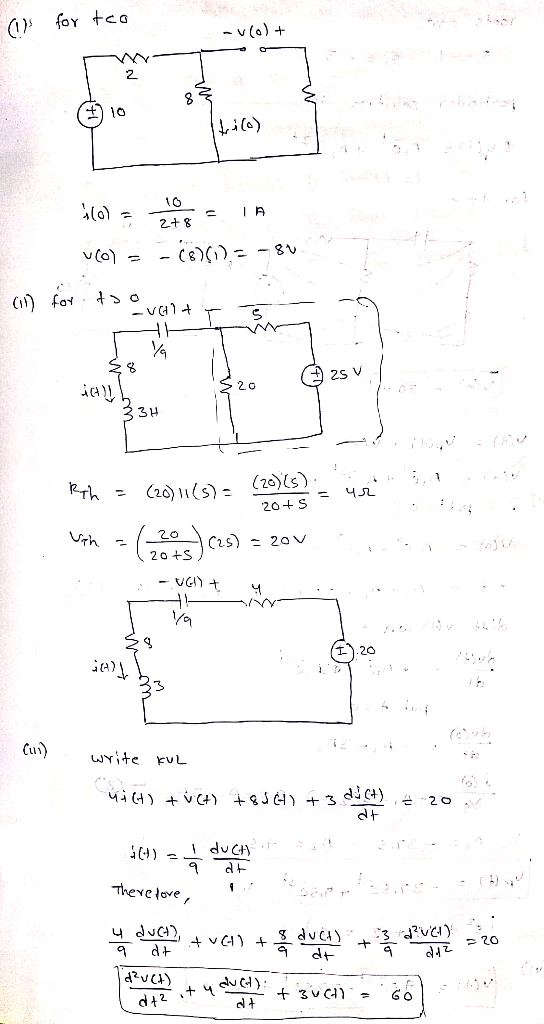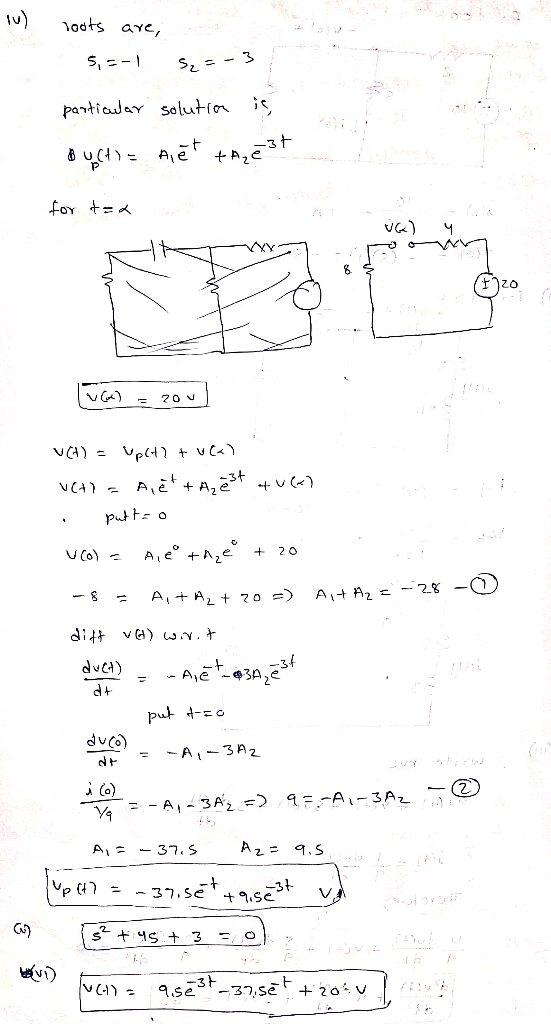##### Add Answer of: Don't use Laplace method Don't use Laplace method Don't use Laplace method Don't use Laplace meth...
Similar Homework Help Questions
• ### Consider the circuit depicted in Fig. 2. The switch SW1 has been closed for a long time before it...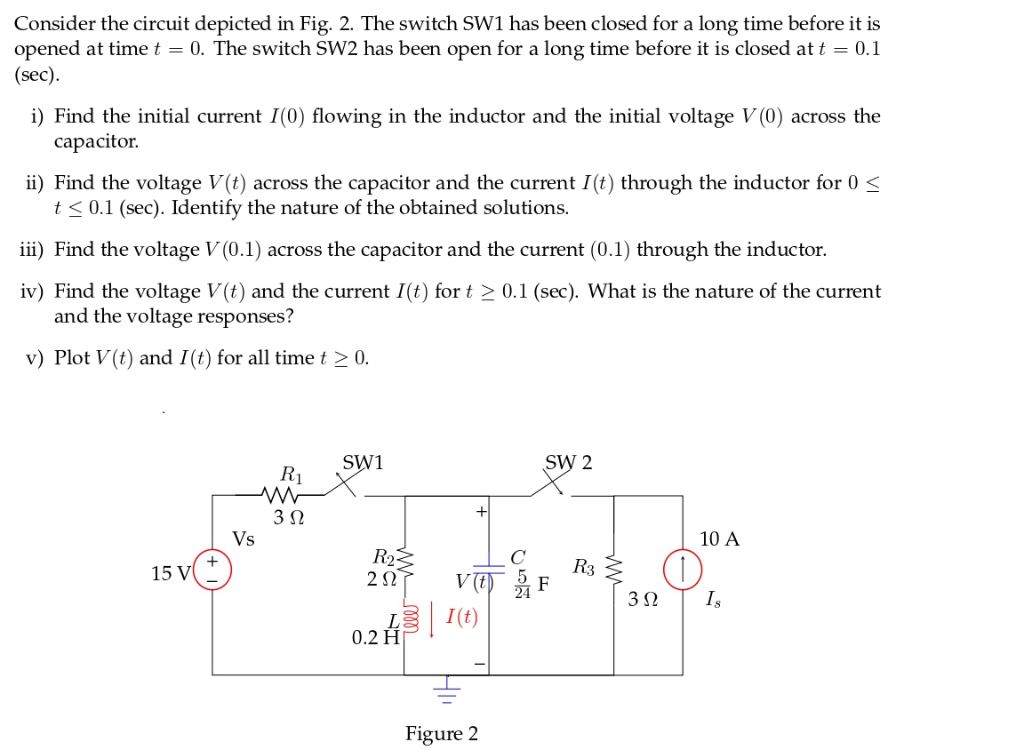Consider the circuit depicted in Fig. 2. The switch SW1 has been closed for a long time before it is opened at time t -0. The switch SW2 has been open for a long time before it is closed att -0.1 sec i) Find the initial current I(0) flowing in the inductor and the initial voltage V (0) across the capacitor ii) Find the voltage V(t) across the capacitor and the current I(t) through the inductor for 0 t <...

• ### do not use s domain method ,use only differential equation 3. In the circuit shown, switch 1 has been closed for a long time before it is opened at t 0, and switch 2 has been opened for a long time b...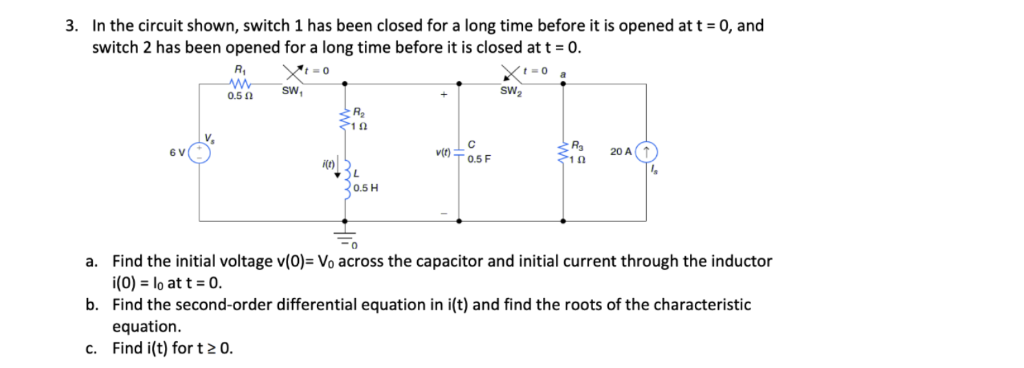do not use s domain method ,use only differential equation 3. In the circuit shown, switch 1 has been closed for a long time before it is opened at t 0, and switch 2 has been opened for a long time before it is closed at t = 0. SW2 sw, 0.5Ω R2 1(2 A, 20 A i(t) 0.5 H a. Find the initial voltage v(O)- Vo across the capacitor and initial current through the inductor (0) lo at t...

• ### SW1 t=0 6uF Given the capacitor circuit, assume SW1 has been closed and SW2 open for...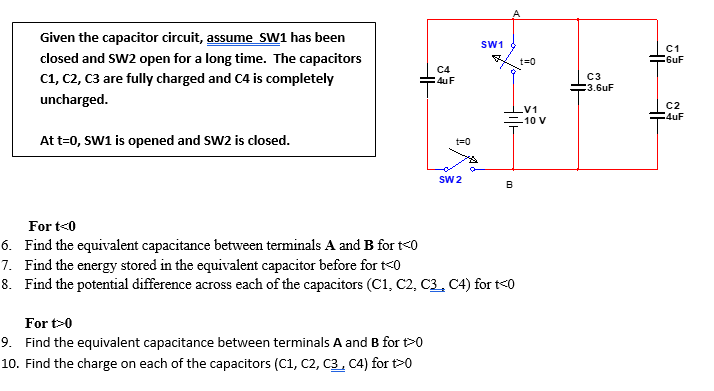SW1 t=0 6uF Given the capacitor circuit, assume SW1 has been closed and SW2 open for a long time. The capacitors C1, C2, C3 are fully charged and C4 is completely uncharged. | 04 4uF C3 3.6uF J C2 - 10 V Auf At t=0, SW1 is opened and sw2 is closed. SW2 For t<0 6. Find the equivalent capacitance between terminals A and B fort<0 7. Find the energy stored in the equivalent capacitor before for t<0 8. Find...

• ### Circuit - CapacitorThe switch in thecircuit below is initially closed. The capacitor begins to chargewhen the switch is opened at t = 0.a. What is the voltage across the capacitor immediately afteropening the switch (t = 0+)?b. Find the voltage across each resistor in the circuit att = 0+.c. Find the current flowing through each resistor att = 0+.d. Find the voltage across each resistor in the circuit att = ∞.e. What voltage does the capacitor charge to if the switch is...

• ### Use electric circuit analysis techniques and Laplace methods to find the charge, q(t), on the...

Use electric circuit analysis techniques and Laplace methods to find the charge, q(t), on the capacitor as a function of time. The switch is closed at t = 0 and theinitial voltage V0 on the capacitor is 3 Volts. The circuit is a series circuit with an open switch, capacitor and resistor all in series.

• ### Problem 7. Perform the following for the circuit below using the source transformation method 40 ...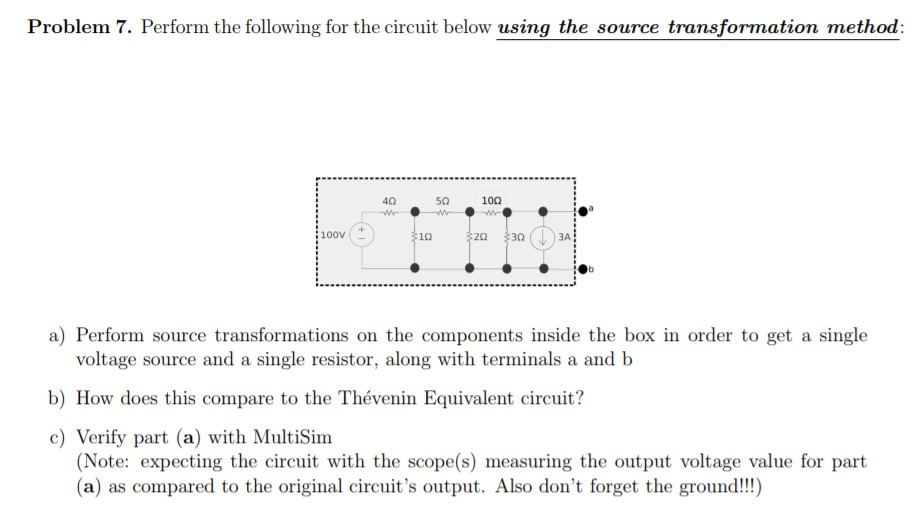Need help with Multisim to simulate these circuit diagrams. Any Multisim works, preferrably Multisim Live Problem 7. Perform the following for the circuit below using the source transformation method 40 5Ω 10Ω 100V 1Ω 20 30 3A a) Perform source transformations on the components inside the box in order to get a single voltage source and a single resistor, along with terminals a and b b) How does this compare to the Thévenin Equivalent circuit? c) Verify part (a) with...

• ### Please do the problem if you can do ALL parts. t-0 a SW1 SW2 0.5 Ω 2 1Ω V. R3 20 A T v(t) 0.5 F 0.5 H 0 Find the initial current i(0) through the inductor and the initial voltage v(0) across the capa...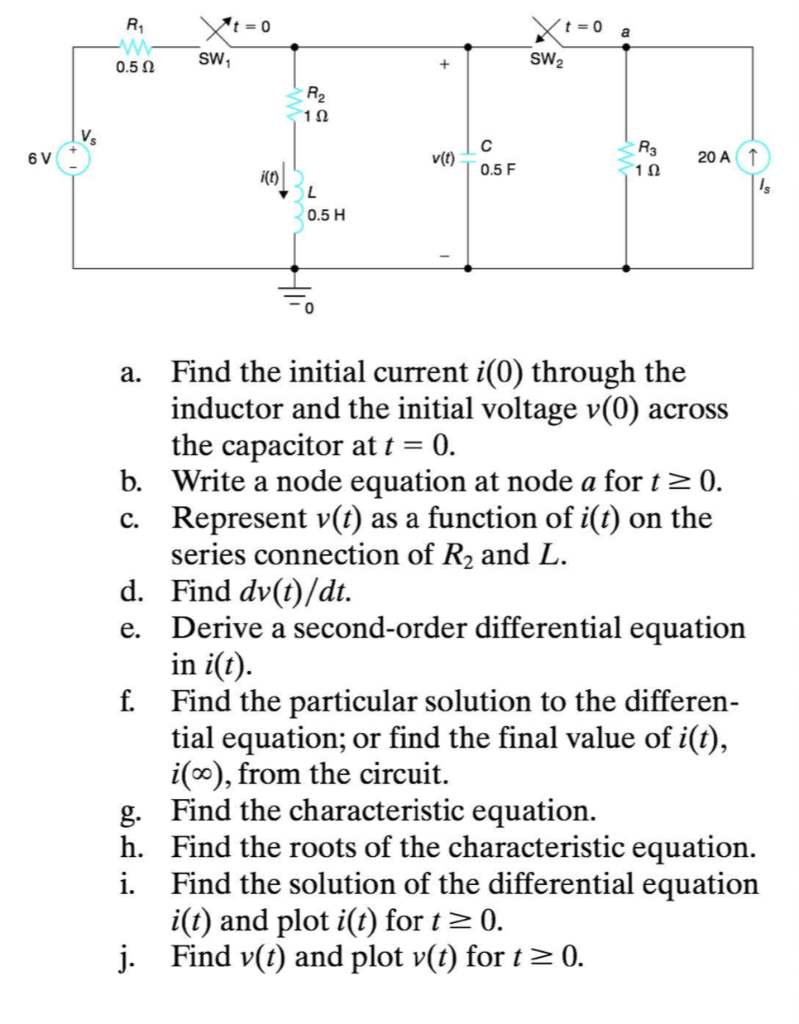Please do the problem if you can do ALL parts. t-0 a SW1 SW2 0.5 Ω 2 1Ω V. R3 20 A T v(t) 0.5 F 0.5 H 0 Find the initial current i(0) through the inductor and the initial voltage v(0) across the capacitor at t 0. b. Write a node equation at node a fort2 0. c. Represent v(t) as a function of i(t) on the series connection of R2 and L. Find dv(t)/dt. Derive a second-order differential...

• ### 1 k2 2 AF 12V 12 V Circuit A Circuit B Figure 3: Figure for Question 3. In Circuit A in Figure 3)...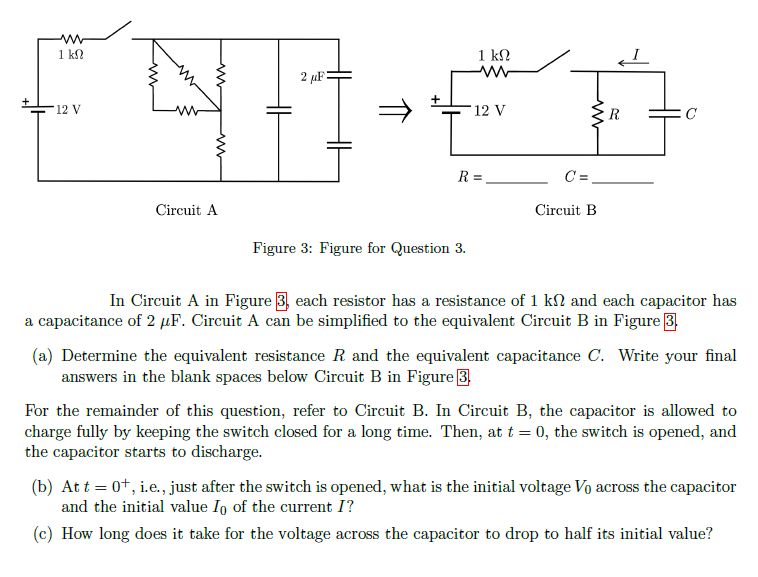1 k2 2 AF 12V 12 V Circuit A Circuit B Figure 3: Figure for Question 3. In Circuit A in Figure 3) each resistor has a resistance of 1 kΩ and each capacitor has a capacitance of 2 μF. Circuit A can be simplified to the equivalent Circuit B in Figure 3 (a) Determine the equivalent resistance R and the equivalent capacitance C. Write your final answers in the blank spaces below Circuit B in Figure 3 For the...

• ### 6: In the circuit shown in Figure-6, input voltage of 15V de was switched ON at t-o. (a) Convert the circuit its Laplace equivalent at t >0, if ILO)-2A and vc (0)-6V. b) Find the capacitor voltage...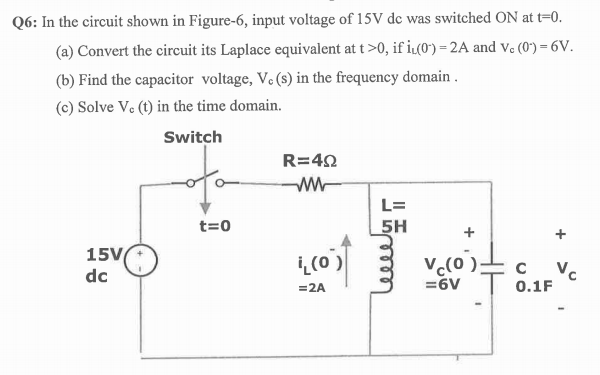6: In the circuit shown in Figure-6, input voltage of 15V de was switched ON at t-o. (a) Convert the circuit its Laplace equivalent at t >0, if ILO)-2A and vc (0)-6V. b) Find the capacitor voltage, Ve (s) in the frequency domain (c) Solve Ve (t) in the time domain. Switch L= 5H t-o 15V (0 ) = 2A V (o =6V 0.1F 6: In the circuit shown in Figure-6, input voltage of 15V de was switched ON at...

• ### Laplace tranform of electrical circuit....NEED URGENT HELP!!!!!Voltage = 12V2 Ressistor = 12OhmCapacitor = (1/170)FInductor = 2HIn the Circuit shown above, the switch is in postion 1 untilthe steady state is achieved.It is moved to position postion 2 att=0.using the laplace transform technique,find and sketch i(t) fort >= 0.

Need Online Homework Help?